# Reverse Engineering a Professional Writeup

## Goal

Take a published data visualization and recreate it step-by-step in R with `ggplot2`. This lab is based on section 3.3 of the textbook Modern Data Science with R.

## Creating a GitHub Repo for This Lab

1. Before editing this file, visit https://classroom.github.com/a/3ZMYrRdk to create a repository on GitHub for this lab. Then create a New RStudio project from the repo (the URL will probably be something like `http://github.com/ocstats/stat209-s2021-lab5-crdawson` but with your account name at the end), and save this file in it. Stage, commit, and push the changes.

## The Source

The visualization we will recreate comes from this FiveThirtyEight post about the historical popularity of various baby names. We will focus on the second plot, depicting how many “Josephs” were born in each year, and how many of those are estimated to be alive today. For convenience, I’ve embedded the plot in the lab below.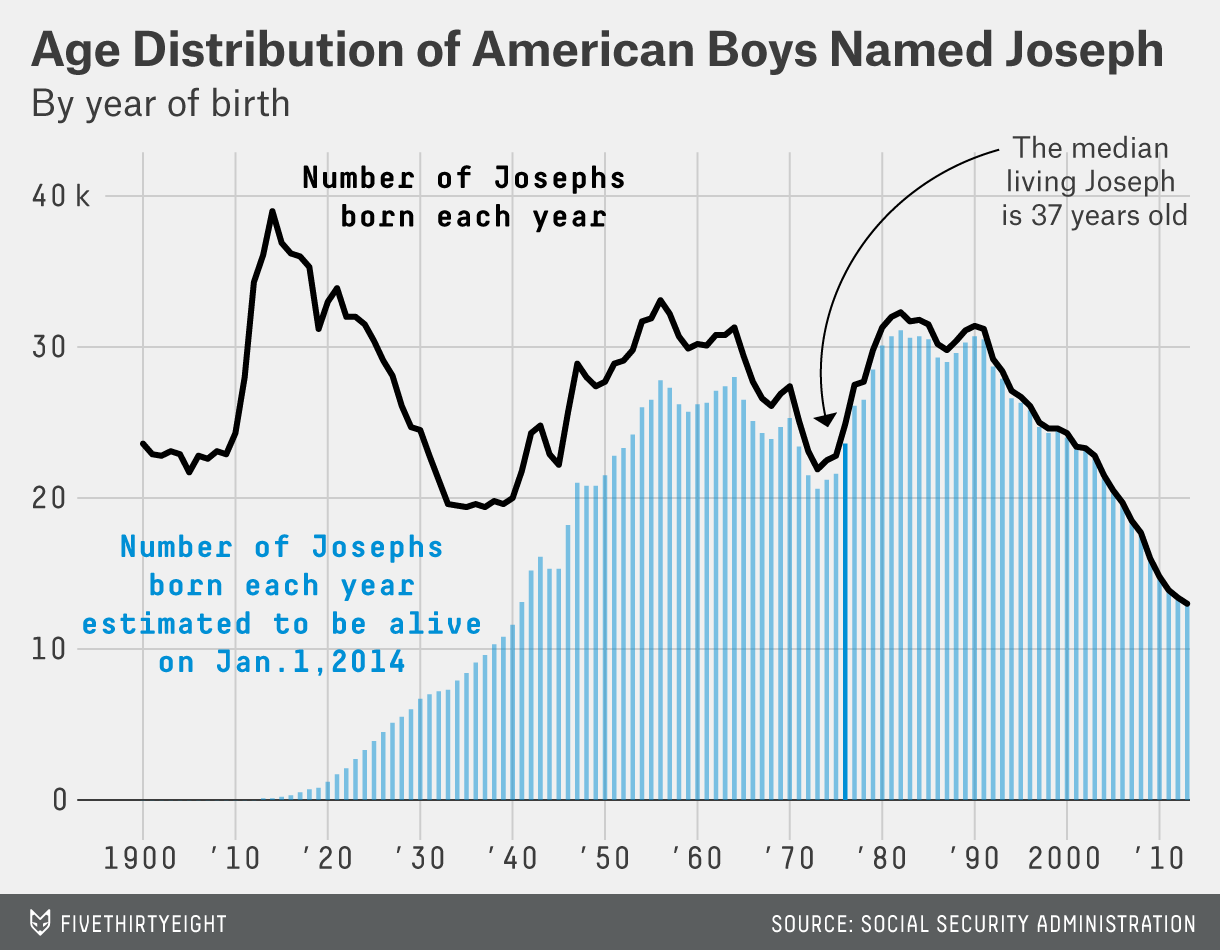1. Before we write any code, examine the plot and write a sentence or two summarizing what it tells us. Then identify the data dimensions (variables) involved, what visual cue(s) each variable is mapped to, what coordinate systems and scales are used.

## Data

The Social Security Administration (SSA) provides historical data on how many babies were born in each year with various names. The relevant data is provided in the `lifetables` data frame from the `babynames` package. Let’s load it now.

Code

``````library(babynames)
data(lifetables)``````

To make life easier, the `mdsr` package provides a function called `make_baby_names_dist()` that does some useful preprocessing of the data so that we don’t have to deal with any data-wrangling yet. It takes no arguments and returns a modified data frame.

Code

``````library(mdsr)
BabynamesDist <- make_babynames_dist()
glimpse(BabynamesDist)``````
``````## Rows: 1,639,722
## Columns: 9
## \$ year            <dbl> 1900, 1900, 1900, 1900, 1900, 1900, 1900, 1900, 1…
## \$ sex             <chr> "F", "F", "F", "F", "F", "F", "F", "F", "F", "F",…
## \$ name            <chr> "Mary", "Helen", "Anna", "Margaret", "Ruth", "Eli…
## \$ n               <int> 16706, 6343, 6114, 5304, 4765, 4096, 3920, 3896, …
## \$ prop            <dbl> 0.05257559, 0.01996211, 0.01924142, 0.01669226, 0…
## \$ alive_prob      <dbl> 0, 0, 0, 0, 0, 0, 0, 0, 0, 0, 0, 0, 0, 0, 0, 0, 0…
## \$ count_thousands <dbl> 16.706, 6.343, 6.114, 5.304, 4.765, 4.096, 3.920,…
## \$ age_today       <dbl> 114, 114, 114, 114, 114, 114, 114, 114, 114, 114,…
## \$ est_alive_today <dbl> 0, 0, 0, 0, 0, 0, 0, 0, 0, 0, 0, 0, 0, 0, 0, 0, 0…``````

## Extracting the data we need

The plot only involves male babies named “Joseph”, so let’s create a filtered dataset with just those births.

Code:

``````Josephs <- BabynamesDist %>%
filter(name == "Joseph", sex == "M")
glimpse(Josephs)``````
``````## Rows: 111
## Columns: 9
## \$ year            <dbl> 1900, 1901, 1902, 1903, 1904, 1905, 1906, 1907, 1…
## \$ sex             <chr> "M", "M", "M", "M", "M", "M", "M", "M", "M", "M",…
## \$ name            <chr> "Joseph", "Joseph", "Joseph", "Joseph", "Joseph",…
## \$ n               <int> 3714, 2766, 3098, 3121, 3291, 3302, 3527, 3844, 4…
## \$ prop            <dbl> 0.02290712, 0.02392837, 0.02333710, 0.02413281, 0…
## \$ alive_prob      <dbl> 0.000000, 0.000025, 0.000050, 0.000075, 0.000100,…
## \$ count_thousands <dbl> 3.714, 2.766, 3.098, 3.121, 3.291, 3.302, 3.527, …
## \$ age_today       <dbl> 114, 113, 112, 111, 110, 109, 108, 107, 106, 105,…
## \$ est_alive_today <dbl> 0.000000, 0.069150, 0.154900, 0.234075, 0.329100,…``````

## Setting up the base plot

There are two `geoms` in this plot: a line depicting the total number of Josephs born in each year, and a set of bars depicting the number estimated to be alive today. In both cases, the x-axis depicts the year; however, there are two different variables mapped to the y-axis. So we don’t want to set up a global mapping to the y-axis; instead we will define the y mapping at the level of each geom. At the global level, we’ll just define the x mapping.

Code

``````joseph_base_plot <- Josephs %>%
ggplot(aes(x = year))
joseph_base_plot``````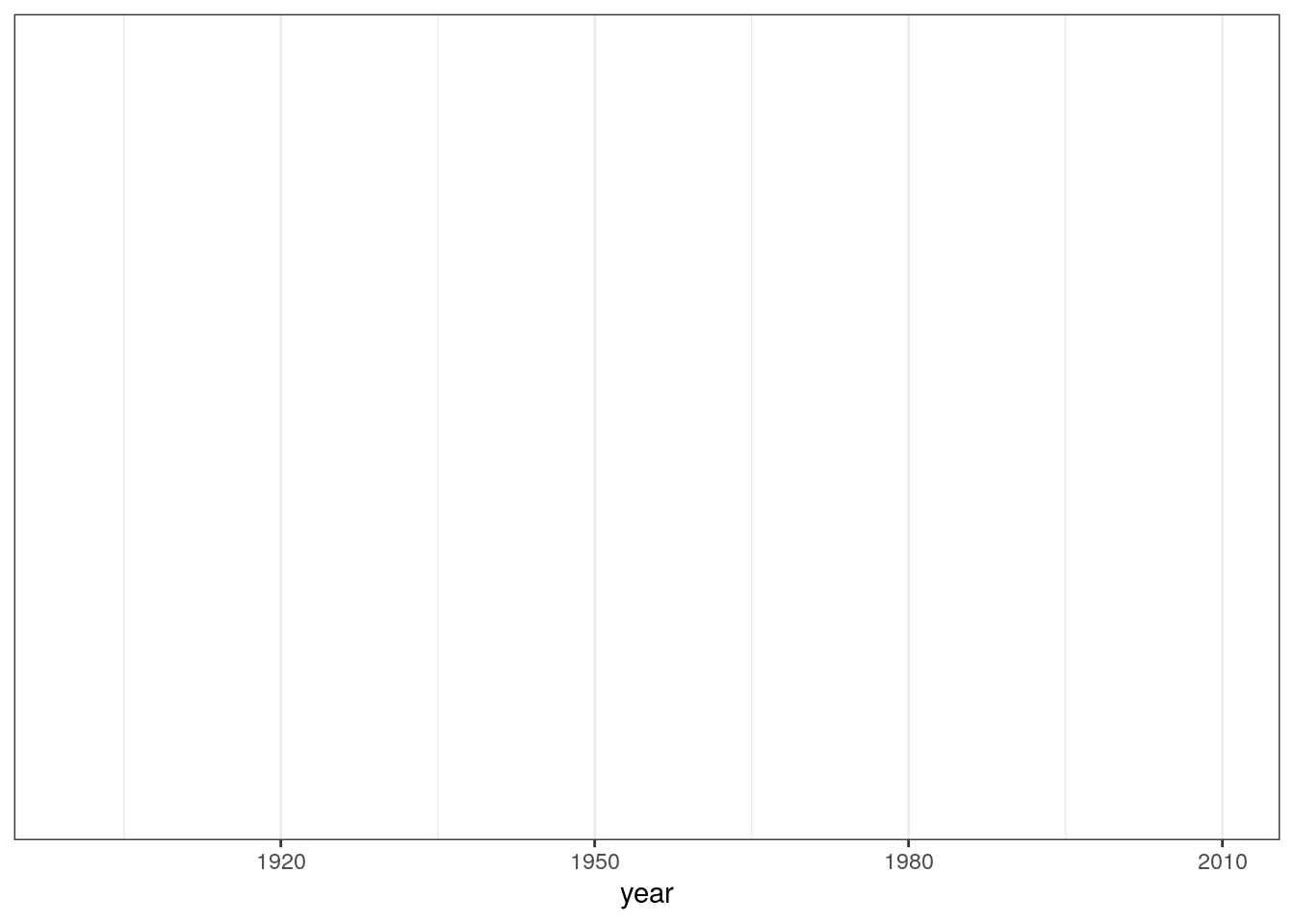## Adding the `geom`s

The bar graph component of the plot is based on the estimated number of male “Joseph”s alive today from each birth year. This is approximately the number of male Josephs born that year (`count_thousands`) times the probability that someone born that year is still alive now (`alive_prob`). The product of these two variables has already been computed for us as `est_alive_today`.

Let’s try adding a `geom_bar()` with the estimated number alive today (`est_alive_today`) as the height of the bars.

Attempted Code:

``````## Will generate an error!
joseph_base_plot + geom_bar(aes(y = est_alive_today))``````

Oops, we get an error message.

The reason this gives an error is that the default for a bar graph is to count how often each level of the “x” variable occurs, and plot that count on the “y” axis. This is what we would want to do if each case in our data was an individual Joseph. In this type of plot, we can’t also map a variable to the y dimension; hence the error.

In our data, each case is a year, and we have the count precomputed in the `est_alive_today` variable.

To tell `geom_bar` to use an existing variable instead of counting cases, we can specify `stat = "identity"`.

Code:

``````joseph_base_plot +
geom_bar(
stat = "identity",
aes(y = est_alive_today))``````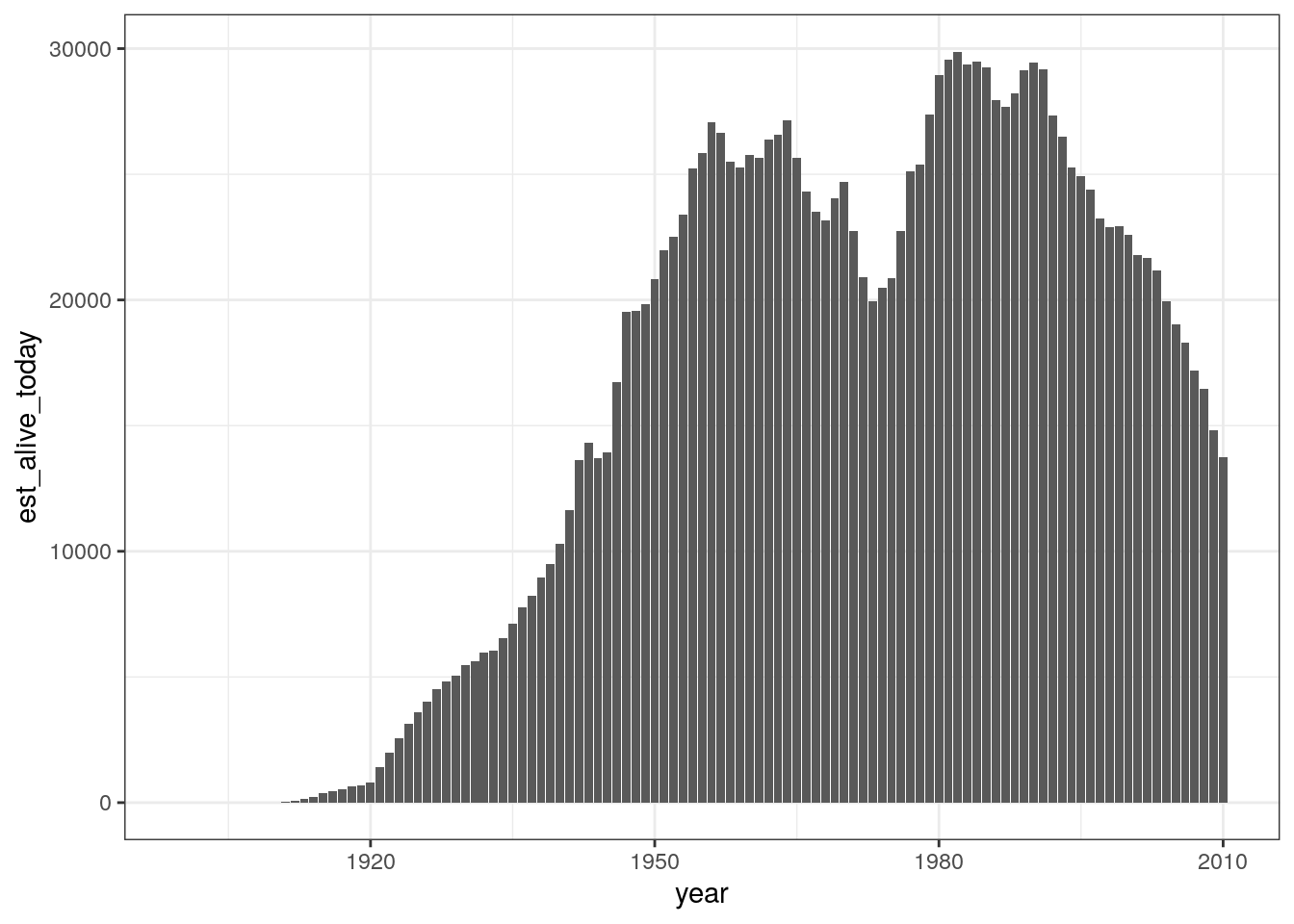Hmm… This shows the data we want, but it’s a little cluttered to have such big numbers on the y axis. The original plot shows thousands on the y axis; let’s do the same.

Code:

``````joseph_base_plot +
geom_bar(
stat = "identity",
aes(y = est_alive_today / 1000))``````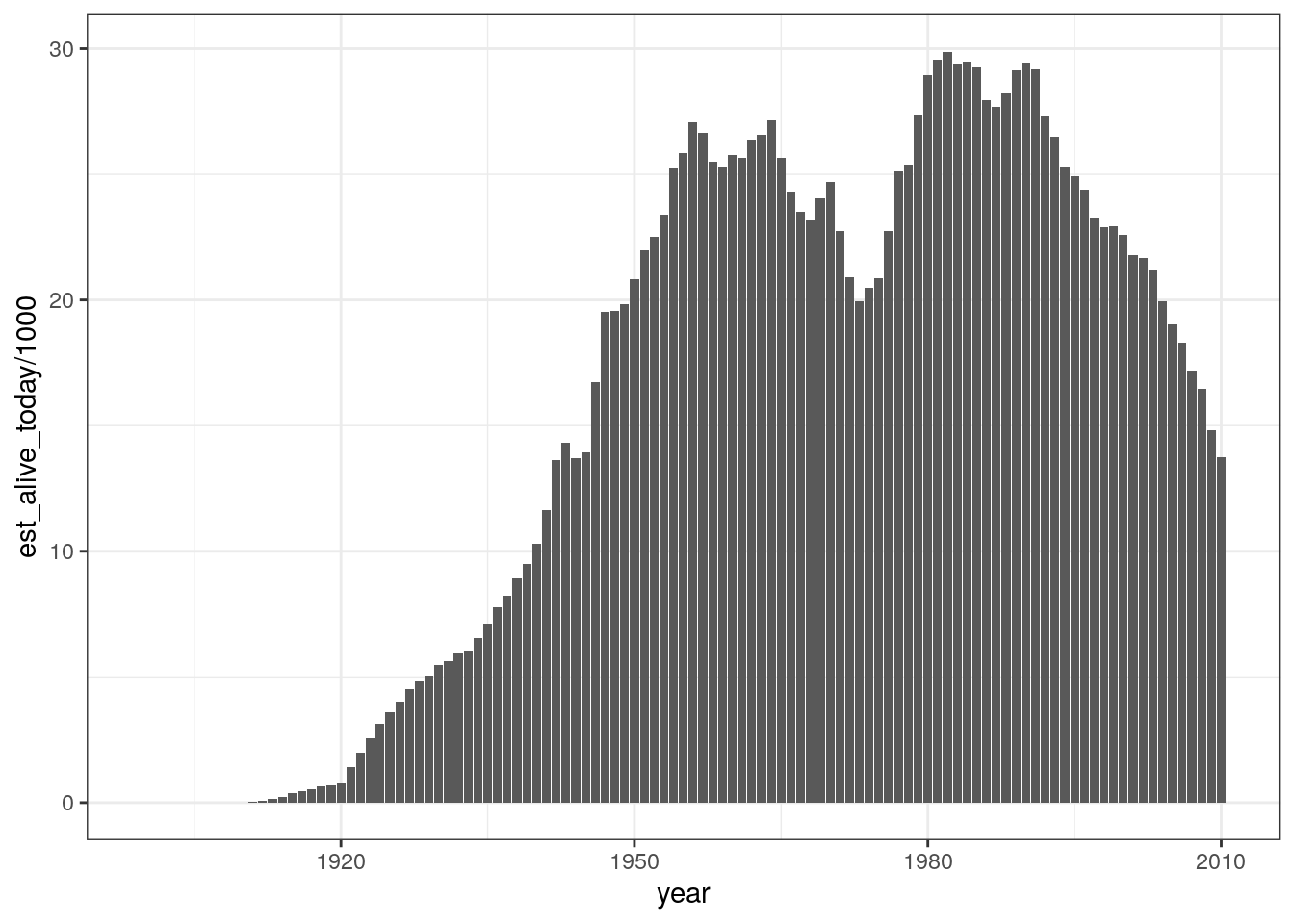That looks cleaner. Let’s try to match the colors of the original bars. The light blue fill is roughly color `#b2d7e9`, and for the white outlines, we’ll just set `color="white"`.

Code:

``````joseph_base_plot +
geom_bar(
stat = "identity",
aes(y = est_alive_today / 1000),
fill = "#b2d7e9",  # fill controls the interior of the bars
color = "white"    # color controls the outline of the bars
)``````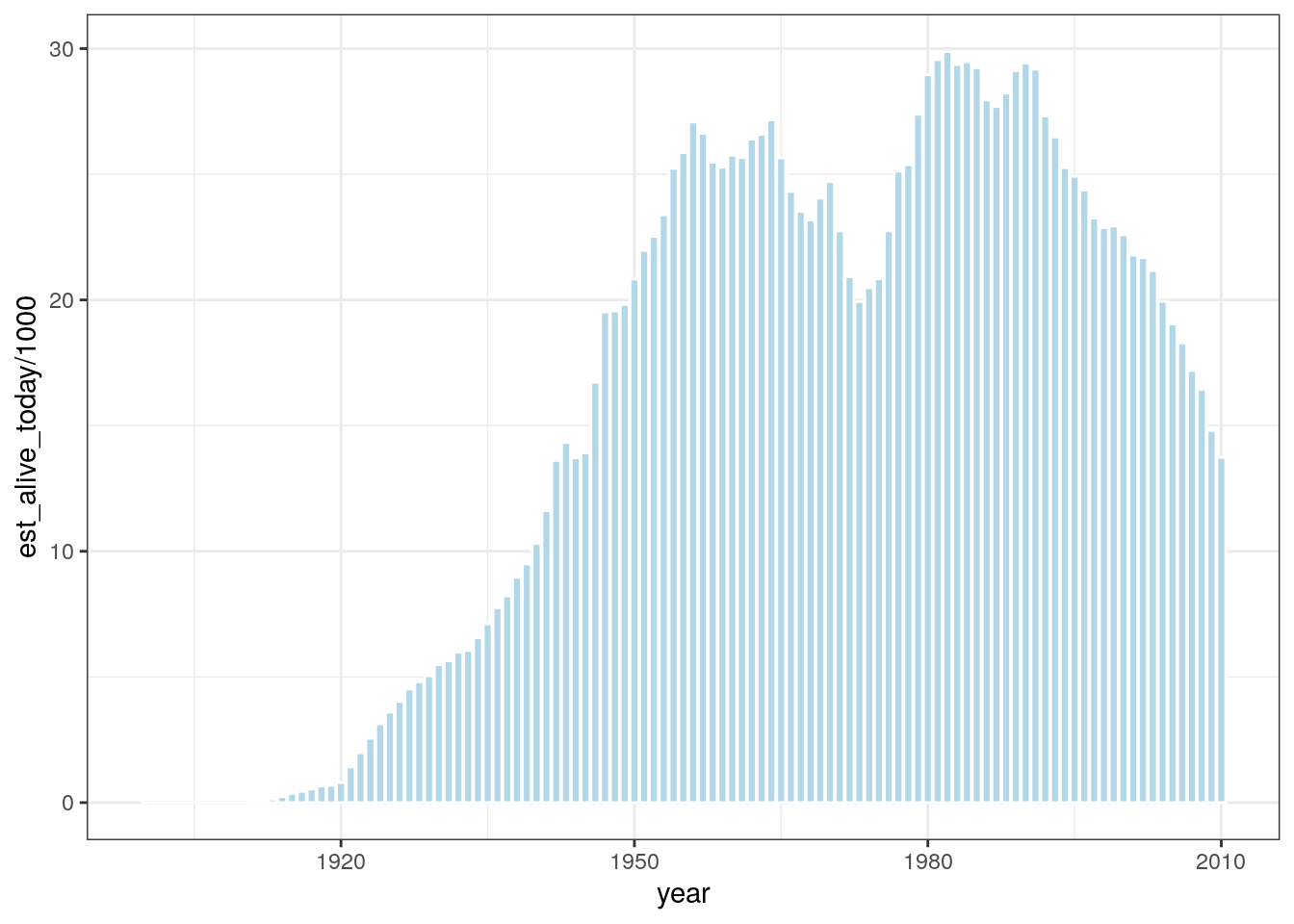Ok, we should be done tinkering with the bar component, so let’s save our updated plot.

Code:

``````joseph_bar_plot <- joseph_base_plot +
geom_bar(
stat = "identity",
aes(y = est_alive_today / 1000),
fill = "#b2d7e9",
color = "white"
)``````

Now let’s add the line. This should depict the total number of births in thousands, which is calculated already in the `count_thousands` variable.

Code:

``````joseph_bar_line_plot <-
joseph_bar_plot +
geom_line(aes(y = count_thousands))
joseph_bar_line_plot``````